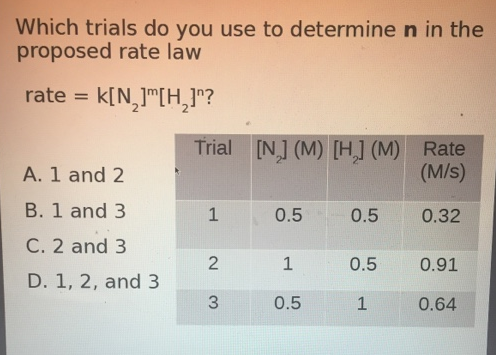# Problem: Which trials do you use to determine n in the proposed rate law rate = k[N2]m [H2]n? a. 1 and 2 b. 1 and 3 c. 2 and 3 d. 1, 2, and 3

###### FREE Expert Solution
93% (494 ratings)###### Problem Details

Which trials do you use to determine n in the proposed rate law

rate = k[N2]m [H2]n

a. 1 and 2

b. 1 and 3

c. 2 and 3

d. 1, 2, and 3